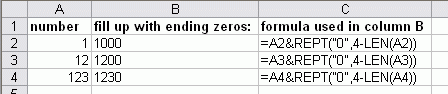Can the "Fill > fill up with leading zero's" option under be made to work on leading or trailing zeros? For instance, if I have three numbers:
1
12
123

I would run the option to fill them with trailing zeros so they would look like:
1000
1200
1230

We want to normalize the numbers so they would all be the same length (123450000 or 123456789) since there weren't the optional 4 digits on all of the entries.

At this moment I don't see a big general purpose for such a tool but I have added it to the wishlist. If you are interested in such an addition to ASAP Utilities, please leave a comment on this post.

Meanwhile, you can achieve this result in just plain Excel by using a combination of the =LEN() and =REPT() functions.
For example the following formula will fill up the value in A2 up with ending zeros to a total length of 4:
`=A2&REPT("0",4-LEN(A2))`As you see in the example the numbers are left-aligned. This is because Excel treats them as 'text' values. If you need to do some calculations on the new numbers with trailing zeros, you can wrap the =VALUE() function around it, which converts a text string that represents a number to a number.
`=VALUE(A2&REPT("0",4-LEN(A2)))`

#### Bastien

Website: https://www.asap-utilities.com